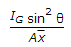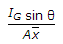# Mechanical Engineering - Hydraulics and Fluid Mechanics

### Exercise :: Hydraulics and Fluid Mechanics - Section 8

1.

Bulk modulus of a fluid is the ratio of

 A. shear stress to shear strain B. increase in volume to the viscosity of fluid C. increase in pressure to the volumetric strain D. critical velocity to the viscosity of fluid

Explanation:

No answer description available for this question. Let us discuss.

2.

The pressure at a point 4 m below the free surface of water is

 A. 19.24 kPa B. 29.24 kPa C. 39.24 kPa D. 49.24 kPa

Explanation:

No answer description available for this question. Let us discuss.

3.

A structure used to dam up a stream or river over which the water flows is called

 A. orifice B. notch C. weir D. dam

Explanation:

No answer description available for this question. Let us discuss.

4.

The coefficient of discharge for an external mouthpiece depends upon

 A. velocity of liquid B. pressure of liquid C. area of mouthpiece D. length of mouthpiece

Explanation:

No answer description available for this question. Let us discuss.

5.

The depth of centre of pressure lor an immersed surface inclined at an angle θ with the liquid surface lies at a distance equal to __________ the centre of gravity.

 A.below B.above C.below D.above